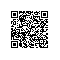# python多线程同步实例分析

python多线程同步实例分析

python多线程同步
python中提供两个标准库thread和threading用于对线程的支持，python3中已放弃对前者的支持，后者是一种更高层次封装的线程库，接下来均以后者为例。

===== 同步关系 =====
Thread A:
write(share_data)
V(S) # 释放资源

Thread B:
P(S) # 获取资源
read(share_data)

===== 互斥关系 =====
Thread A:
Lock.acquire(); # 获得锁
write(share_data)
Lock.release() # 释放锁

Thread C:
Lock.acquire(); # 获得锁
write(share_data)
Lock.release() # 释放锁

share_data = 0

def tstart(arg):

time.sleep(0.1)
global share_data
for i in xrange(1000):
share_data += 1


if name == '__main__':

t1 = threading.Thread(target = tstart, args = ('',))
t2 = threading.Thread(target = tstart, args = ('',))
t1.start()
t2.start()
t1.join()
t2.join()
print 'share_data result:', share_data

View Code

View Code

threading模块的给python线程提供了一些同步机制，具体用法可以参照官网上的文档说明。

Lock：互斥锁，只能有一个线程获取，获取该锁的线程才能执行，否则阻塞；
RLock：递归锁，也称可重入锁，已经获得该锁的线程可以继续多次获得该锁，而不会被阻塞，释放的次数必须和获取的次数相同才会真正释放该锁；
Condition：条件变量，使得一个线程等待另一个线程满足特定条件，比如改变状态或某个值。然后会主动通知另一个线程，并主动放弃锁；
Semaphore：信号锁。为线程间共享的有限资源提供一个”计数器”，如果没有可用资源则会被阻塞；
Event：事件锁，任意数量的线程等待某个事件的发生，在该事件发生后所有线程被激活；
Timer：一种计时器（其用法比较简单，不算同步机制暂不介绍）

import threading
import time
lock = threading.Lock() # step 1: 创建互斥锁
share_data = 0

def tstart(arg):

time.sleep(0.1)
global share_data
if lock.acquire():       # step 2: 获取互斥锁，否则阻塞当前线程
share_data += 1
lock.release()          # step 3: 释放互斥锁


if name == '__main__':

tlst = list()
for i in xrange(10):
t = threading.Thread(target=tstart, args=('',))
tlst.append(t)
for t in tlst:
t.start()
tlst.join()
print("This is main function at：%s" % time.time())
print 'share_data result:', share_data

This is main function at：1564909315.86
share_data result: 7

import threading
import time
rlock = threading.RLock() # step 1: 创建重入锁
share_data = 0

def check_data():

global share_data
if rlock.acquire():
if share_data > 10:
share_data = 0
rlock.release()


def tstart(arg):

time.sleep(0.1)
global share_data
if rlock.acquire():       # step 2: 获取重入锁，否则阻塞当前线程
check_data()
share_data += 1
rlock.release()          # step 3: 释放重入锁


if name == '__main__':

t1 = threading.Thread(target = tstart, args = ('',))
t1.start()
t1.join()
print("This is main function at：%s" % time.time())
print 'share_data result:', share_data

acquire(即P操作)
release(即V操作)

def get_wait_time():

return random.random()/5.0


# 资源数0

S = threading.Semaphore(0)
def consumer(name):

S.acquire()
time.sleep(get_wait_time())
print name


def producer(name):

# time.sleep(0.1)
time.sleep(get_wait_time())
print name
S.release()


if name == "__main__":

for i in xrange(5, 10):
c = threading.Thread(target=consumer, args=("consumer:%s"%i, ))
c.start()
for i in xrange(5):
p = threading.Thread(target=producer, args=("producer:%s"%i, ))
p.start()
time.sleep(2)

View Code

def get_time():

return time.strftime("%Y-%m-%d %H:%M:%S")


def show_start_info(tname):

print '%s start at: %s' %(tname, get_time())


def show_acquire_info(tname):

print '%s acquire at: %s' % (tname, time.time())


def show_add_once_res(tname):

print '%s add: %s at: %s' % (tname, share_data, time.time())


def show_end_info(tname):

print 'End %s with: %s at: %s' % (tname, share_data, time.time())


def show_wait_info(tname):

print '%s wait at: %s' % (tname, time.time())

Python文档给出的说明如下：

Note: an awakened thread does not actually return from its wait() call until it can reacquire the lock. Since notify() does not release the lock, its caller should.

share_data, max_len = '#', 6
cond = threading.Condition()

def addA(tname):

show_start_info(tname)
cond.acquire()
time.sleep(1)
show_acquire_info(tname)
global share_data
while len(share_data) <= max_len:
if share_data[-1] != 'A':
share_data += 'A'
time.sleep(1)
cond.notify()
show_add_once_res(tname)
else:
# show_wait_info(tname)
cond.wait()
cond.release()
show_end_info(tname)


def addB(tname):

show_start_info(tname)
cond.acquire()
time.sleep(1)
show_acquire_info(tname)
global share_data
while len(share_data) <= max_len:
if share_data[-1] != 'B':
share_data += 'B'
time.sleep(1)
cond.notify()
show_add_once_res(tname)
else:
# show_wait_info(tname)
cond.wait()
cond.release()
show_end_info(tname)


if name == "__main__":

t1 = threading.Thread(target=addA, args=("Thread 1", ))
t2 = threading.Thread(target=addB, args=("Thread 2", ))
t1.start()
t2.start()
t1.join()
t2.join()
print "share_data:", share_data

View Code

E = threading.Event()
E.clear()
res1, res2, cnt, lst = 0, 0, 3, ("player2", 'both', 'player1')

def show_round_res():

print ("Stop! judging... %s win!" % lst[cmp(res1, res2) + 1])


def judge():

global cnt
while cnt > 0:
print 'start game!'
E.set()
time.sleep(1)
E.clear()
show_round_res()
time.sleep(1)
cnt -= 1
print "game over by judge!"


def player1():

global res1
while cnt > 0:
if E.is_set():
res1 = random.randint(1, 6)
print "player1 get %d" % res1
time.sleep(1.5)
E.wait()
print "player1 quit!"


def player2():

global res2
while cnt > 0:
if E.is_set():
res2 = random.randint(1, 6)
print "player2 get %d" % res2
time.sleep(1.5)
E.wait()
print "player2 quit!"


if name == "__main__":

t1 = threading.Thread(target=judge, args=( ))
t2 = threading.Thread(target=player1, args=( ))
t3 = threading.Thread(target=player2, args=( ))
t1.start()
t2.start()
t3.start()
t1.join()
E.set()

View Code使用钉钉扫一扫加入圈子
+ 订阅